# Fourier Series in Signal and System

## The Concept of Fourier Series

To understand the concept of Fourier series we first need to understand the concept of a signal. A signal is something that has information (Sound signal, Video Signal etc.) and can further be classified basically as AC and DC signal according to their characteristics.

### Frequency

The frequency of a signal is also a very important thing that we need to understand. Frequency is a way of expressing how many times a certain point of a signal has occurred during a period of 1 second).  The conventional unit of frequency was Cycles per second and was later changed to Hertz. The unit Cycles per second is more intuitive to understand the concept of frequency as shown in the diagrams below.

Take a look at figure 1, we have one cycle in the period of 1 second hence frequency is 1Hz(Hertz).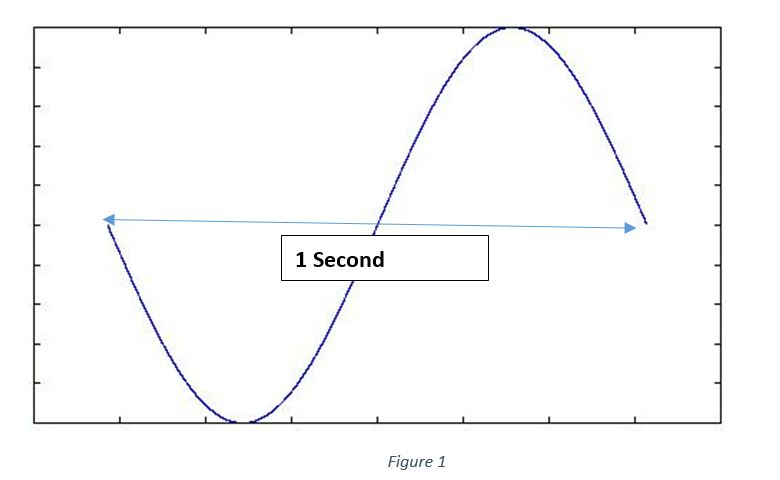In figure 2 we have two cycles in the period of 1 second hence we say the signal has frequncy of 2 Hz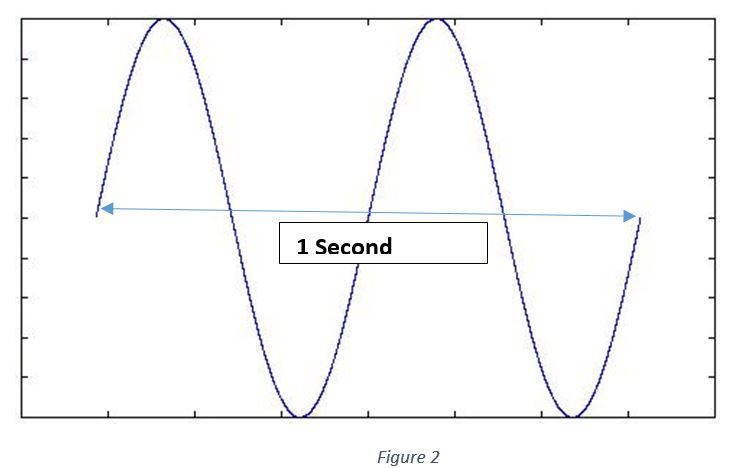### Harmonics

As we just saw the concept of frequency we also need to know something called as Harmonics. Suppose if we consider any signal (for simplicity let’s consider 1Hz) now any signal with a frequency of integer multiple of 1Hz is known as harmonic of 1Hz.

Considered signal – 1Hz

2 x 1Hz = 2Hz (This is the second Harmonic of 1Hz)

3 x 1Hz = 3Hz (This is the third Harmonic of 1Hz)

…….

Considered signal – 5Hz

2 x 5Hz = 10Hz (This is the second Harmonic of 5Hz)

3 x 5Hz = 15Hz (This is the third Harmonic of 5Hz)

…….

Now we are equipped with the basic knowledge of signal, frequency and harmonics and it is time to dive into the world of Fourier analysis.

### The Fourier Series

Jean B. Joseph Fourier was a French mathematician who proposed an idea that any periodic signal can be represented by addition of scaled basis signals of different frequencies(harmonics). The idea behind the statement is very simple, take any periodic signal (A signal which repeats itself after certain amount of time) and we can approximate that signal by adding scaled signals of specific type with different frequencies. The concept has an analogy with the concepts of vector resolution which says Any vector can be represented by its horizontal and vertical components.

The concepts of periodic waveform and Fourier series statement can be clarified by following diagrams.

As it can be seen in figure 3, The green part of the waveform repeats itself again and again and hence the green part is known as period and the original waveform is known as periodic waveform.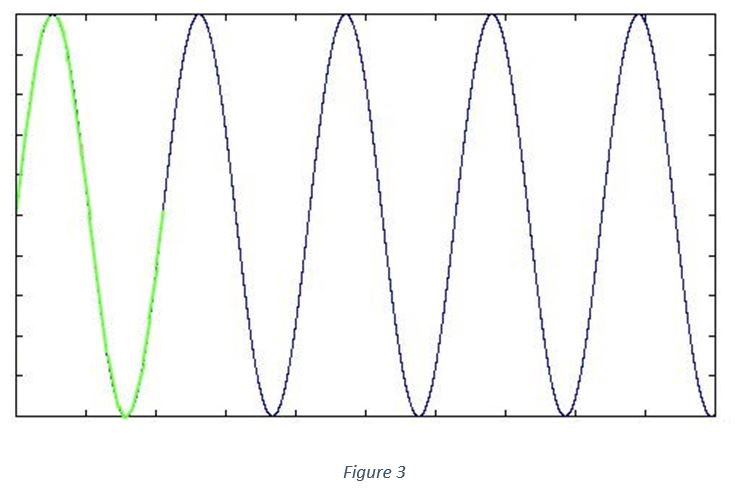The figure 4 shows how to interpret the statement of Fourier Series, a basis signal(Orange) scaled with Coefficients(Green) is added together to get approximated periodic signal(Blue).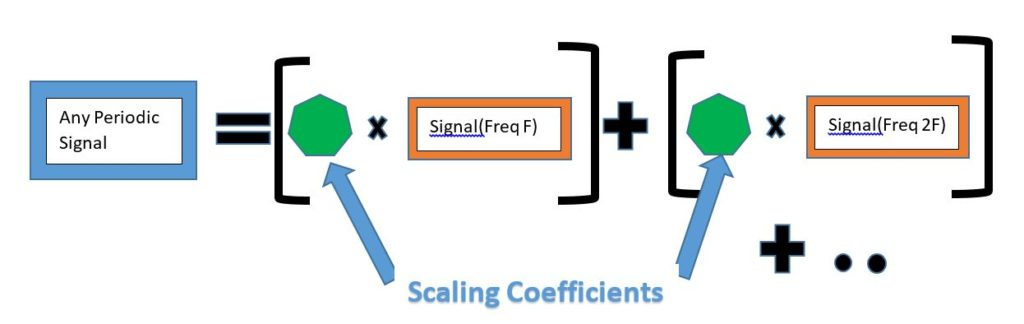The basis signals that are used to approximate the periodic signal shown in the diagram (Orange) are important as those are the signals which will be scaled and added together to get our periodic signal.

The type of Fourier series is governed by the type of basis signals used for approximation. If we consider basis signals as complex exponentials, then the Fourier Series is known as Exponential Fourier Series.    If the basis signals are sines and cosines then the Fourier Series is known as Trigonometric Fourier Series. (Note that exponential and trigonometric Fourier series are just different representation of same concept and can be converted from one form to another at any time)

As a part of introduction we will only look at Trigonometric Fourier Series in this article. A formal mathematical equation for Trigonometric Fourier Series is as follows.Where f(x) is the function /signal that we want to approximate, and a(n) and b(n) are the scaling coefficients which are also known as Fourier Coefficients are given bySo the Trigonometric Fourier Series says that any periodic function/signal can be expressed as addition of scaled sins and cosines having different frequencies(harmonics).

We can understand this by considering our voice, as we know human voice is a mixed frequency signal so if we consider a human voice sample function then we can tell using Fourier series that what are the frequencies (of basis signals) that are needed to approximate the human voice sample. In other words, the Fourier series gives us what are the frequency contents in the considered signal.

Wait!!!…. so for this example of human voice… if I take basis signals and add them together with proper scaling can I generate human voice????

YES, that is what the Fourier series is providing, you can consider these basis signals as components of the original signal and while solving the Fourier series we are actually resolving that original signal into its components, just like the vectors.

To actually get a feel for the Fourier Series we need to solve an example. The classic example is a square wave, consider a square wave as shown in figure 4 below. The square wave has an amplitude of +c in the period 0 to pi and an amplitude of -c in the period -pi to 0.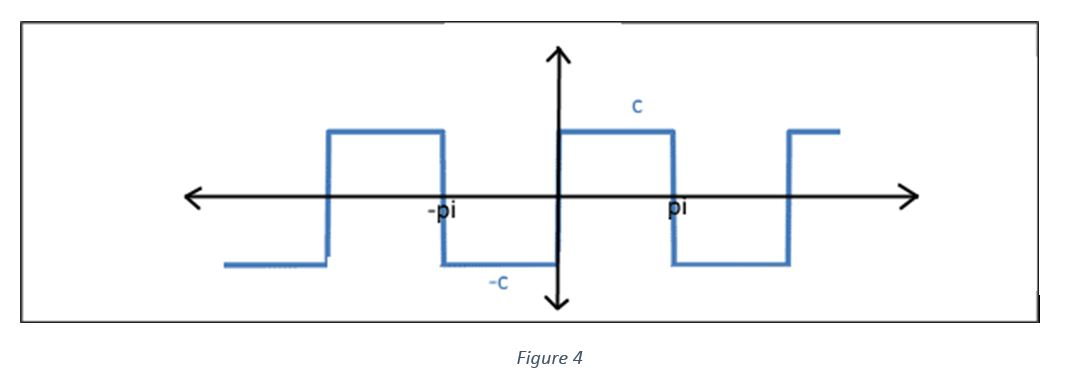Let’s identify the period of the waveform (find the portion of the waveform that is repeating itself again and again) , The period is from -pi to pi. (Take a look at figure  5 for clarification green color is representing one period of the waveform)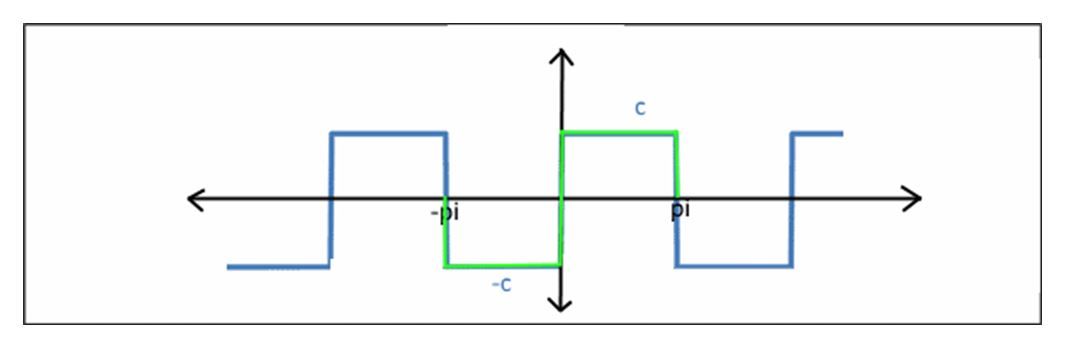Now to solve mathematically we need to find value of L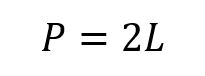From figure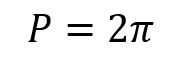HenceLet us calculate coefficients,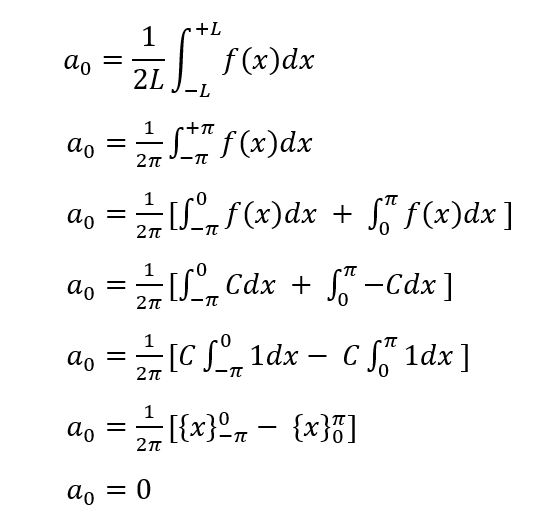Now a(n)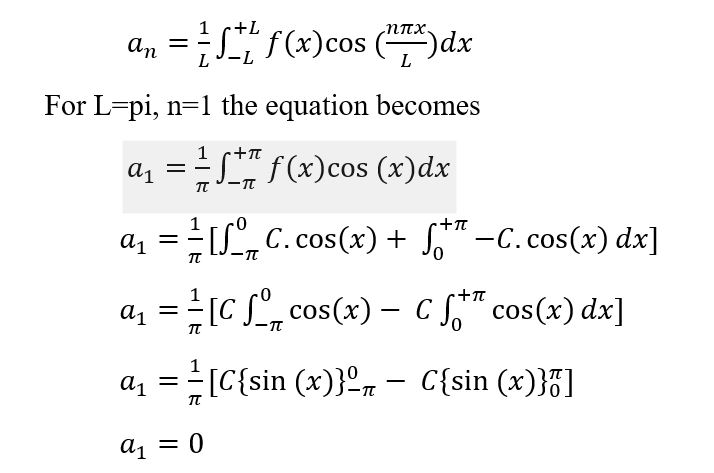Similarly n=2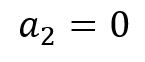For n=3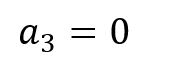Now b(n)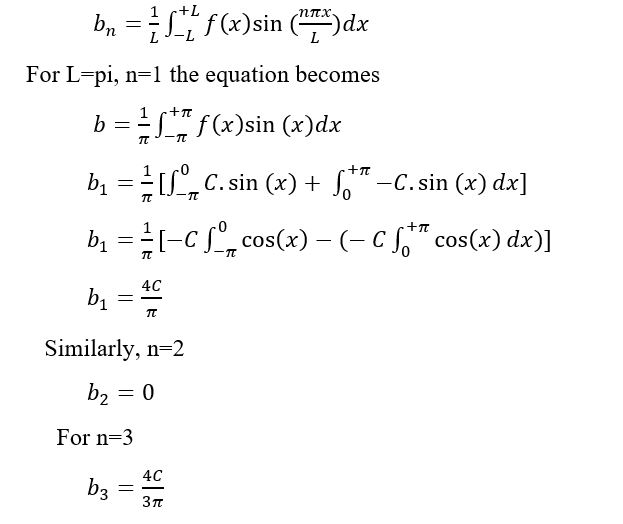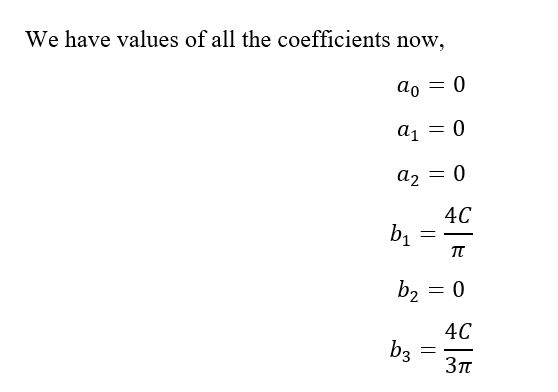Note that we can calculate coefficients for n=4,5,6…. But for the purpose of understanding we are only calculating 3 coefficients.

Remember our series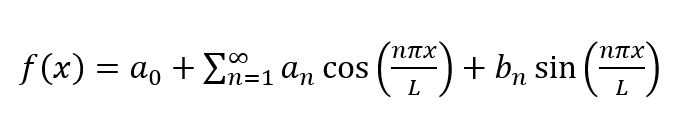After putting the values of coefficients we get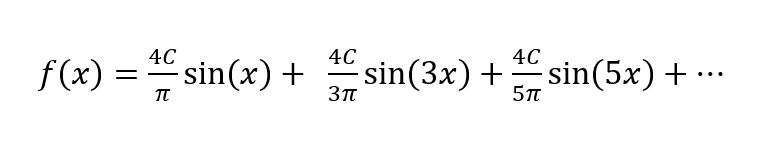So as I promised you we have resolved the original function into its components of harmonics. Now I said that if we take these basis (sines and cosines) signals and add them together we will get the square wave back…. let’s check it out

Note that for adding these signals together I am making some assumptions, consider coefficient part  to be 1 for simplicity as it is nothing but a constant.

Now the series becomes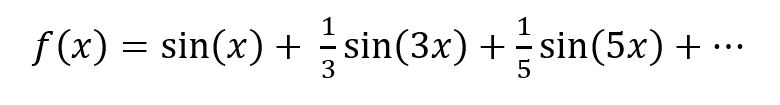sin(x) is represented below in figure 5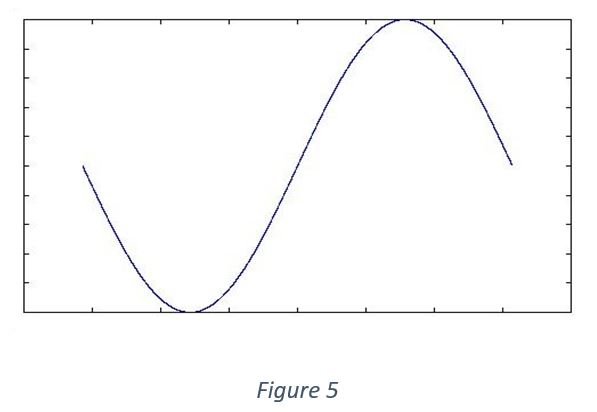(1/3)*sin(3x) is represented below in figure 6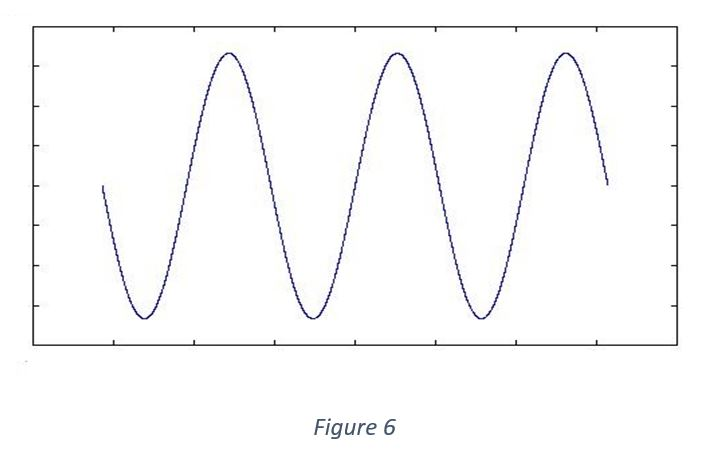(1/7)*sin(5x) is represented below in figure 7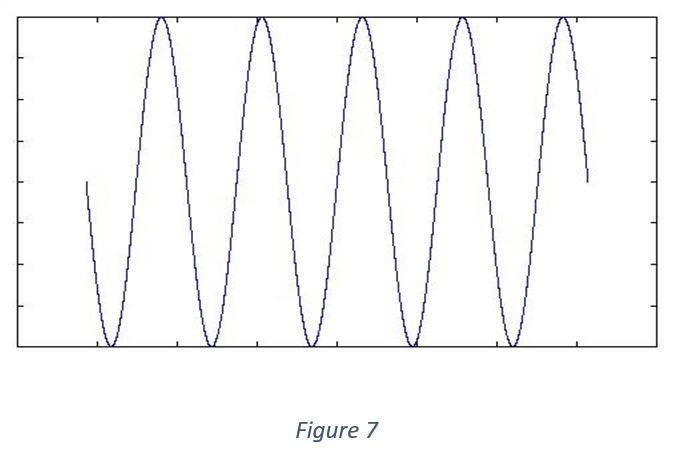Now lets add these signals….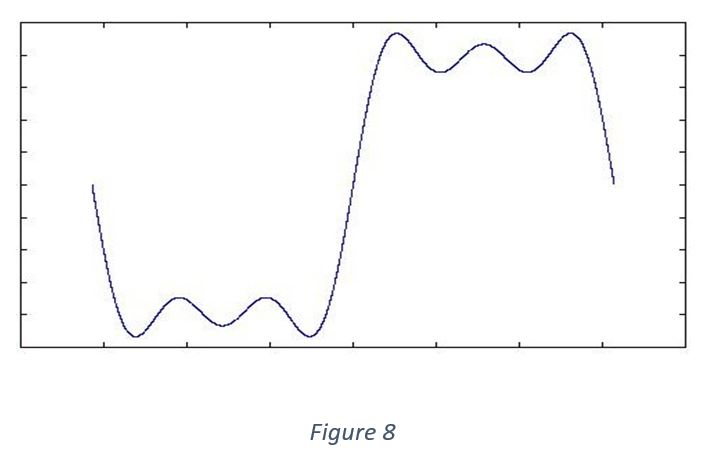The Wave already looks like a square wave, as we add more and more number of harmonics the waveform will become more and more squarish.